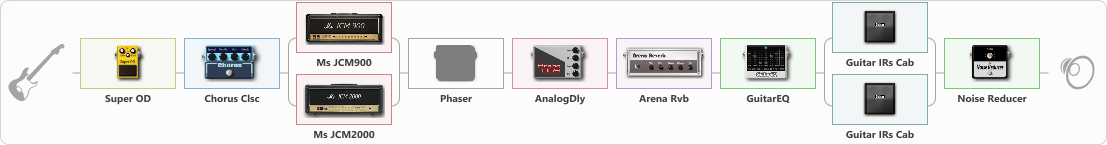# Marshall Mix

Discussion in 'ToneLib-GFX presets' started by Tenarus, Feb 28, 2020.

1. Marshall Mix

Preset name: Marshall Mix

To get a sound similar to Nuno Bettencourt with a Musicman EVH

Effects chain:Effect: "Super OD" (Overdrive / Distortion), active - "yes"
{
"Drive" = 28
"Tone" = 88
"Level" = 24
}

Effect: "Chorus Clsc" (Modulation / Sfx), active - "yes"
{
"Speed" = 3.5
"Depth" = 58
"Center" = 1.0
"Mode" = Stereo
}

Effect: "Splitter" (Dynamics / Filter), active - "yes"
{
"A-Bypass" = Off
"A-Pan" = 0
"A-Level" = 55
"B-Bypass" = Off
"B-Pan" = 0
"B-Level" = 55

'A' branch:
{

Effect: "Ms JCM900" (Amp simulators), active - "yes"
{
"Gain" = 60
"Bass" = 58
"Middle" = 42
"Treble" = 66
"Presence" = 62
"Master" = 50
"Level (dB)" = 0
}
}
'B' branch:
{

Effect: "Ms JCM2000" (Amp simulators), active - "yes"
{
"Gain" = 62
"Bass" = 58
"Middle" = 42
"Treble" = 66
"Presence" = 62
"Master" = 50
"Level (dB)" = 0
}
}
}

Effect: "Phaser" (Modulation / Sfx), active - "no"
{
"Model" = MXR Phase 90
"Speed" = 1.9
"Depth" = 36
"Reso" = 26
"Freq" = 5.0
}

Effect: "AnalogDly" (Delay), active - "yes"
{
"Time" = 380
"Feedback" = 0
"Tone" = 70
"Mix" = 50
}

Effect: "Arena Rvb" (Reverb), active - "yes"
{
"Time" = 7.3
"PreDelay" = 23
"LoDamp" = 18
"HiDamp" = 21
"Mix" = 43
}

Effect: "GuitarEQ" (Dynamics / Filter), active - "yes"
{
"160 Hz" = 1
"400 Hz" = 5
"800 Hz" = 5
"1.6 kHz" = 2
"3.2 kHz" = 2
"6.4 kHz" = 6
"12 kHz" = 6
"Level (dB)" = 0
}

Effect: "Splitter" (Dynamics / Filter), active - "yes"
{
"A-Bypass" = Off
"A-Pan" = 0
"A-Level" = 55
"B-Bypass" = Off
"B-Pan" = 0
"B-Level" = 55

'A' branch:
{

Effect: "Guitar IRs Cab" (Cabinets), active - "yes"
{
"Model" = Peavey 5150 (4x12")
"Mic Position" = Center
"Mic Distance" = Near
"Low Cut (Hz)" = 154
"Hi Cut (kHz)" = 8.5
"Mix" = 100
"Level (dB)" = 0
}
}
'B' branch:
{

Effect: "Guitar IRs Cab" (Cabinets), active - "yes"
{
"Model" = Peavey 5150 (4x12")
"Mic Position" = Center
"Mic Distance" = Near
"Low Cut (Hz)" = 100
"Hi Cut (kHz)" = 5.5
"Mix" = 100
"Level (dB)" = 0
}
}
}

Effect: "Noise Reducer" (Dynamics / Filter), active - "yes"
{
"Sens" = 66
"Mode" = Soft
}

Note: You will need to download and install the ToneLib-GFX software to use the preset.

#### Attached Files:

• ###### Marshall_Mix.tlgfx
File size:
1.3 KB
Views:
3,217
spesial72, emre and enzo ohata like this.